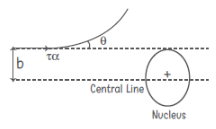# Atoms Class 12 Notes Physics Chapter 12 - CBSE

## What are Atoms ?

 Model Important Points Limitations Alpha particle Scattering experiment This experiment led Rutherford to discovery of atomic nucleus.Most of the space in atom is empty.N(θ) ∝(1/sin4(θ/2))where N(θ) = number of α particlesθ = scattered angle Rutherford’s Atomic Model According to this model, the entire positive charge of the atom and almost its entire mass is concentrated in a small region of the atom. Rutherford named this region as the nucleus. The nucleus is surrounded by electrons. The atom as a whole is neutral, the total negative charge of electrons is equal to the positive charge on the nucleons.For the stability of the atom, Rutherford suggested that the electrons are revolving circular orbits at high speeds. It could not explain the line spectrum of H-atom.It could not explain the stability of atoms. As per Rutherford’s model electrons revolve around the nucleus in a circular path. But particles in motion on a circular path would undergo acceleration and acceleration causes radiation of energy by charged particles. Eventually lose energy and ultimately fall into the nucleus. Bohr’s Atomic Model Bohr incorporated following postulates in his theory:(i)The electron in an atom could revolve in certain stable orbits without the emission of radiant energy.(ii)The electron revolves around the nucleus only in those orbits for which angular momentum is some integral multiple of (h/2π) , where h is Planck constant( = 6.6 × 10–34 Js)L = mvr = n(h/2π) where n = 1, 2, 3 and is called principal quantum number.(iii)Emission of radiation taken place when an electron makes a transition from a higher orbit to a lower orbit. During this transition, a photon is emitted having energy equal to the energy difference between the initial and final states hv = Ei – Ef Bohr model is applicable only to hydrogen like atoms.Bohr model is also unable to explain relative intensities of the frequencies emitted by the hydrogen like atoms.
 Topic/Term Formula Symbol Representation Important Points Rydberg formula for Spectrum of Hydrogen Atom =(1/λ)=[(1/n2f)- (1/n2i)] v= Wave numberR = Rydberg constant= 1.097 × 107 m–1ni = Initial statenF = Final state When the electron in a hydrogen atom jumps from higher energy level to lower energy level, the difference of energies of the two energy levels is emitted as radiation of particular wavelength, known as spectral lines.Spectral lines of different wavelength constitute spectral series such as Lyman, Balmer, Paschen, Brackett and Pfund series. Relation between Impact parameter (b) and scattering angle θ b =(Ze2 cot(θ /2)/(4πε0K)) Z = atomic number of the nucleouse = charge on electronθ = scattering angleK = kinetic energy of the α-particle i.e. (1/2)mv2Distance of closest approach (r0) r0 =(Ze(2e)/4πε0 × K) Z = atomic numbere = charge on electronK = kinetic energy At the distance of closest approach whole of the kinetic energy the alpha particle is converted into potential energy. Energy of hydrogen atoms E = (-e2/8πε0r) e = charge of electron r = radius